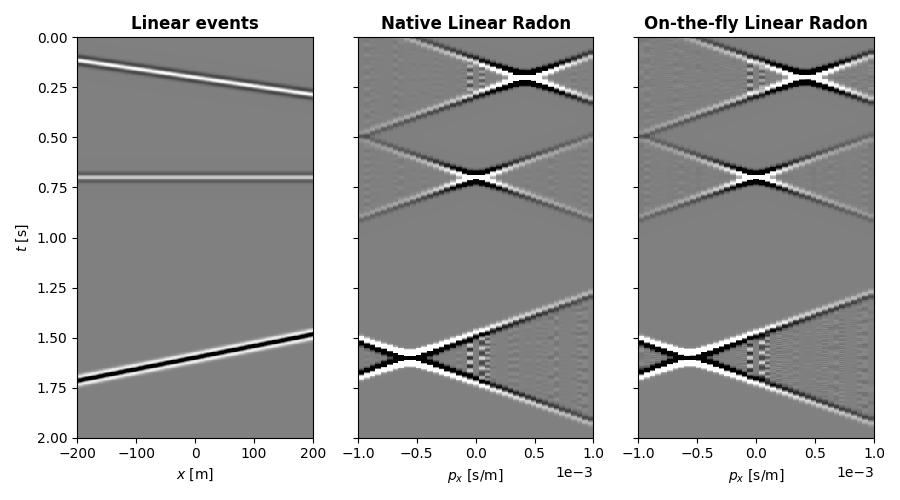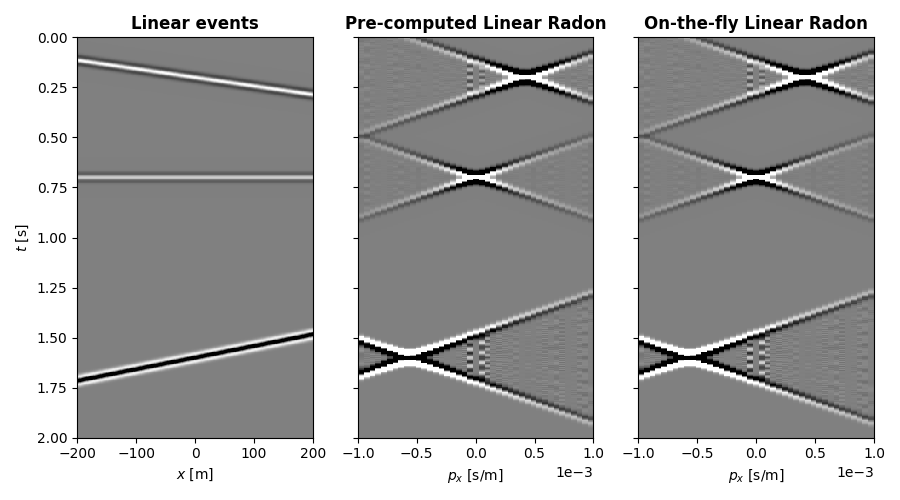This example focuses on the pylops.basicoperators.Spread operator, which is a highly versatile operator in PyLops to perform spreading/stacking operations in a vectorized manner (or efficiently via Numba-jitted for loops).

The pylops.basicoperators.Spread is powerful in its generality, but it may not be obvious for at first how to structure your code to leverage it properly. While it is highly recommended for advanced users to inspect the pylops.signalprocessing.Radon2D and pylops.signalprocessing.Radon3D operators since they are built using the pylops.basicoperators.Spread class, here we provide a simple example on how to get started.

In this example we will recreate a simplified version of the famous linear Radon operator, which stacks data along straight lines with a given intercept and slope.

import matplotlib.pyplot as plt
import numpy as np

import pylops

plt.close("all")


Let’s first define the time and space axes as well as some auxiliary input parameters that we will use to create a Ricker wavelet

par = {
"ox": -200,
"dx": 2,
"nx": 201,
"ot": 0,
"dt": 0.004,
"nt": 501,
"f0": 20,
"nfmax": 210,
}

# Create axis
t, _, x, _ = pylops.utils.seismicevents.makeaxis(par)

# Create centered Ricker wavelet
t_wav = np.arange(41) * par["dt"]
wav, _, _ = pylops.utils.wavelets.ricker(t_wav, f0=par["f0"])


We will create a 2d data with a number of crossing linear events, to which we will later apply our Radon transforms. We use the convenience function pylops.utils.seismicevents.linear2d.

v = 1500  # m/s
t0 = [0.2, 0.7, 1.6]  # seconds
theta = [40, 0, -60]  # degrees
amp = [1.0, 0.6, -2.0]

mlin, mlinwav = pylops.utils.seismicevents.linear2d(x, t, v, t0, theta, amp, wav)


Let’s now define the slowness axis and use pylops.signalprocessing.Radon2D to implement our benchmark linear Radon. Refer to the documentation of the operator for a more detailed mathematical description of linear Radon. Note that pxmax is in s/m, which explains the small value. Its highest value corresponds to the lowest value of velocity in the transform. In this case we choose that to be 1000 m/s.

npx, pxmax = 41, 1e-3
px = np.linspace(-pxmax, pxmax, npx)

t, x, px, centeredh=False, kind="linear", interp=False, engine="numpy"
)

mlinwavR = RLop.H * mlinwav


Now, let’s try to reimplement this operator from scratch using pylops.basicoperators.Spread. Using the on-the-fly approach, and we need to create a function which takes indices of the model domain, here $$(p_x, t_0)$$ where $$p_x$$ is the slope and $$t_0$$ is the intercept of the parametric curve $$t(x) = t_0 + p_x x$$ we wish to spread the model over in the data domain. The function must return an array of size nx, containing the indices corresponding to $$t(x)$$.

The on-the-fly approach is useful when storing the indices in RAM may exhaust resources, especially when computing the indices is fast. When there is enough memory to store the full table of indices (an array of size $$n_x \times n_t \times n_{p_x}$$) the pylops.basicoperators.Spread operator can be used with tables instead. We will see an example of this later.

Returning to our on-the-fly example, we need to create a function which only depends on ipx and it0, so we create a closure around it with all our other auxiliary variables.

def create_radon_fh(xaxis, taxis, pxaxis):
ot = taxis
dt = taxis - taxis
nt = len(taxis)

def fh(ipx, it0):
tx = t[it0] + xaxis * pxaxis[ipx]
it0_frac = (tx - ot) / dt
itx = np.rint(it0_frac)
# Indices outside time axis set to nan
itx[np.isin(itx, range(nt), invert=True)] = np.nan
return itx

return fh

mlinwavROTF = ROTFOp.H * mlinwav


Compare the results between the native Radon transform and the one using our on-the-fly pylops.basicoperators.Spread.

fig, axs = plt.subplots(1, 3, figsize=(9, 5), sharey=True)
axs.imshow(
mlinwav.T,
aspect="auto",
interpolation="nearest",
vmin=-1,
vmax=1,
cmap="gray",
extent=(x.min(), x.max(), t.max(), t.min()),
)
axs.set_title("Linear events", fontsize=12, fontweight="bold")
axs.set_xlabel(r"$x$ [m]")
axs.set_ylabel(r"$t$ [s]")
axs.imshow(
mlinwavR.T,
aspect="auto",
interpolation="nearest",
vmin=-10,
vmax=10,
cmap="gray",
extent=(px.min(), px.max(), t.max(), t.min()),
)
axs.set_xlabel(r"$p_x$ [s/m]")
axs.ticklabel_format(style="sci", axis="x", scilimits=(0, 0))

axs.imshow(
mlinwavROTF.T,
aspect="auto",
interpolation="nearest",
vmin=-10,
vmax=10,
cmap="gray",
extent=(px.min(), px.max(), t.max(), t.min()),
)
axs.set_xlabel(r"$p_x$ [s/m]")
axs.ticklabel_format(style="sci", axis="x", scilimits=(0, 0))
fig.tight_layout()Finally, we will re-implement the example above using pre-computed tables. This is useful when fh is expensive to compute, or requires manual edition prior to usage.

Using a table instead of a function is simple, we just need to apply fh to all our points and store the results.

def create_table(npx, nt, nx):
table = np.full((npx, nt, nx), fill_value=np.nan)
for ipx in range(npx):
for it0 in range(nt):
table[ipx, it0, :] = fRad(ipx, it0)
return table

table = create_table(npx, par["nt"], par["nx"])
RPCOp = pylops.Spread((npx, par["nt"]), (par["nx"], par["nt"]), table=table)

mlinwavRPC = RPCOp.H * mlinwav


Compare the results between the pre-computed or on-the-fly Radon transforms

fig, axs = plt.subplots(1, 3, figsize=(9, 5), sharey=True)
axs.imshow(
mlinwav.T,
aspect="auto",
interpolation="nearest",
vmin=-1,
vmax=1,
cmap="gray",
extent=(x.min(), x.max(), t.max(), t.min()),
)
axs.set_title("Linear events", fontsize=12, fontweight="bold")
axs.set_xlabel(r"$x$ [m]")
axs.set_ylabel(r"$t$ [s]")
axs.imshow(
mlinwavRPC.T,
aspect="auto",
interpolation="nearest",
vmin=-10,
vmax=10,
cmap="gray",
extent=(px.min(), px.max(), t.max(), t.min()),
)
axs.set_xlabel(r"$p_x$ [s/m]")
axs.ticklabel_format(style="sci", axis="x", scilimits=(0, 0))

axs.imshow(
mlinwavROTF.T,
aspect="auto",
interpolation="nearest",
vmin=-10,
vmax=10,
cmap="gray",
extent=(px.min(), px.max(), t.max(), t.min()),
)
axs.set_xlabel(r"$p_x$ [s/m]")